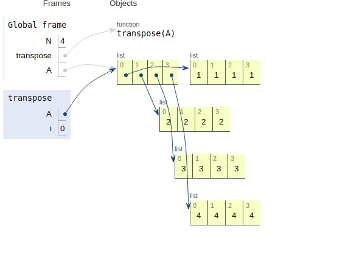# Python Program to find the transpose of a matrix

In this article, we will learn about the solution and approach to solve the given problem statement.

## Problem statement

Given a matrix, we need to store the transpose in the same matrix and display it.

Transpose of a matrix is obtained by changing rows to columns and columns to rows. In other words, transpose of A matrix is obtained by changing A[i][j] to A[j][i].

Let’s see the implementation given below −

## Example

N = 4
def transpose(A):
for i in range(N):
for j in range(i+1, N):
A[i][j], A[j][i] = A[j][i], A[i][j]
# driver code
A = [ [1, 1, 1, 1],
[2, 2, 2, 2],
[3, 3, 3, 3],
[4, 4, 4, 4]]
transpose(A)
print("Modified matrix is")
for i in range(N):
for j in range(N):
print(A[i][j], " ", end='')
print()

## Output

Modified matrix is
1 2 3 4
1 2 3 4
1 2 3 4
1 2 3 4

All the variables and functions are declared in the global scope as shown below −